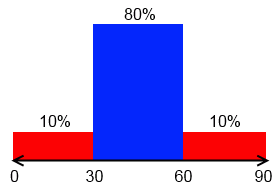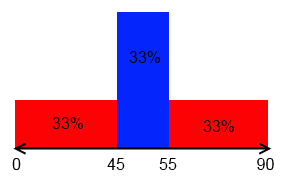# ML Wiki

## Data Discretization

What if we want to transform a continuous attribute to a categorical?

## Equal-Width Partitioning

Also called distance partitioning

• want to divide $X = (x_1, ..., x_m)$ into $N$ equal intervals
• let $A = \min X$ and $B = \max X$
• width: $W = \cfrac{B - A}{N}$
•• suppose that in one such partition you have all your data
• you'll lose a lot of information
• so it's sensible to Outliers

## Equal-Depth Partitioning

Also called frequency partitioning

• Divides $X$ into $N$ intervals,
• with each interval containing approximately same number of samples
• not sensible to outliers
• distribution of values is taken into account
•## Entropy-Based Discretization

Uses entropy to find the best way to split your data

• find the value $\alpha$ that maximizes the Information Gain
• split by $\alpha$
• repeat recursively until have $N$ intervals or no information gain is possible